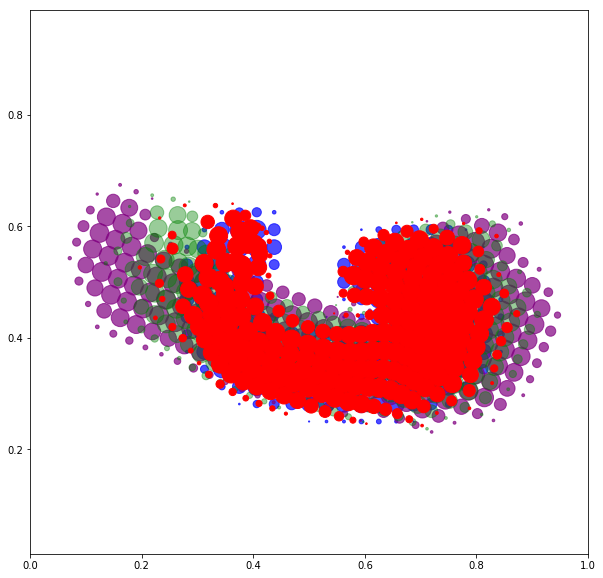# 2) Enforcing the preservation of topology¶

In :
%matplotlib inline
import matplotlib.pyplot as plt

# Mandatory imports...
import numpy as np
import torch
from torch import tensor
from torch.nn import Parameter, ParameterList
from torch.autograd import grad

# Custom modules:
from model import Model  # Optimization blackbox
from display import plot, train_and_display
from torch_complex import rot # Rotations in 2D


We've seen how to work with unlabeled segmentation masks encoded as measures $\alpha$ and $\beta$: the key idea here is to use a data fidelity term which is well-defined up to resampling.

In :
def scal( α, f ) :
"Scalar product between two vectors."
return torch.dot( α.view(-1), f.view(-1) )

def sqdistances(x_i, y_j) :
"Matrix of squared distances, C_ij = |x_i-y_j|^2."
return ( (x_i.unsqueeze(1) - y_j.unsqueeze(0)) ** 2).sum(2)

def distances(x_i, y_j) :
"Matrix of distances, C_ij = |x_i-y_j|^2."
return (x_i.unsqueeze(1) - y_j.unsqueeze(0)).norm(p=2,dim=2)

def fidelity(α, β) :
"Energy Distance between two sampled probability measures."
α_i, x_i = α
β_j, y_j = β
K_xx = -distances(x_i, x_i)
K_xy = -distances(x_i, y_j)
K_yy = -distances(y_j, y_j)
cost = .5*scal( α_i, K_xx @ α_i ) \
-    scal( α_i, K_xy @ β_j ) \
+ .5*scal( β_j, K_yy @ β_j )
return cost


Most often, we work with measures that have been rigidly aligned with each other:

In :
class IsometricRegistration(Model) :
"Find the optimal translation and rotation."
def __init__(self, α) :
"Defines the parameters of a rigid deformation of α."
super(Model, self).__init__()
self.α, self.x = α.detach(), α.detach()
self.θ = Parameter(tensor(  0.     )) # Angle
self.τ = Parameter(tensor( [0.,0.] )) # Position

def __call__(self, t=1.) :
# At the moment, PyTorch does not support complex numbers...
x_t = rot(self.x, t*self.θ) + t*self.τ
return self.α, x_t

def cost(self, target) :
"Returns a cost to optimize."
return fidelity( self(), target)

In :
from sampling import load_measure

α_0 = load_measure("data/hippo_a.png")
β   = load_measure("data/hippo_b.png")
print("Number of atoms : {} and {}.".format(len(α_0), len(β)))

# In practice, we often quotient out affine deformations:
isom = IsometricRegistration(α_0)
isom.fit(β)
α = isom()
α = α.detach().requires_grad_(), α.detach().requires_grad_()

# Let's display everyone:
plt.figure(figsize=(10,10))
plot(β,   "blue",   alpha=.7)
plot(α_0, "purple", alpha=.2)
plot(α,   "red",    alpha=.7)
plt.axis("equal")
plt.axis([0,1,0,1])
plt.show()

Number of atoms : 252 and 182.
It  1:0.028. It  2:0.009. It  3:0.008. It  4:0.008. It  5:0.008.
It  6:0.008. It  7:0.008. It  8:0.008. It  9:0.007. It 10:0.007.
It 11:0.006. It 12:0.006. It 13:0.006. It 14:0.006. It 15:0.007.
It 16:0.006.
b'CONVERGENCE: REL_REDUCTION_OF_F_<=_FACTR*EPSMCH'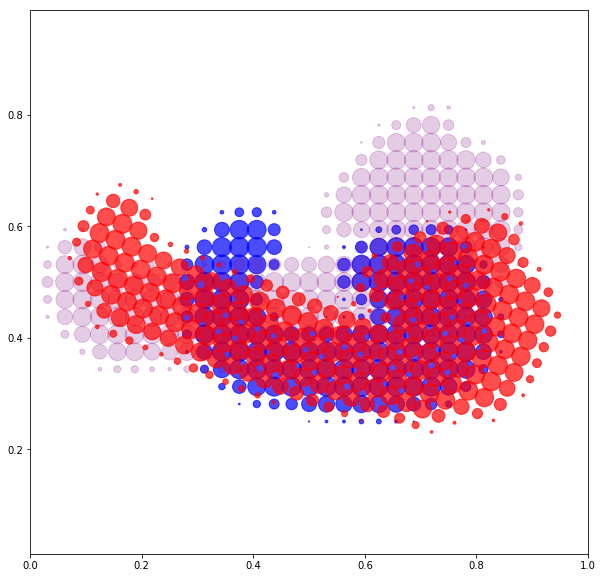## A) Linear models¶

Let us now present some weakly parametrized models that let us study the variability between $\alpha$ and $\beta$ in a continuous way. In the previous notebook, we've seen that the "free particles" setting could be implemented as follows - with control variables $(p_i)\in\mathbb{R}^{N\times 2}$:

In :
class L2Registration(Model) :
"Find the optimal mass positions."
def __init__(self, α) :
"Defines the parameters of a free deformation of α."
super(Model, self).__init__()
self.α, self.x = α.detach(), α.detach()
self.p = Parameter(torch.zeros_like(self.x))

def __call__(self, t=1.) :
"Applies the model on the source point cloud."
x_t =  self.x + t*self.p
return self.α, x_t

def cost(self, target) :
"Returns a cost to optimize."
return fidelity( self(), target)

l2_reg = train_and_display(L2Registration, α, β)

It  1:0.006. It  2:0.005. It  3:0.001. It  4:0.001. It  5:0.000.
It  6:0.000. It  7:0.000. It  8:0.000. It  9:0.000. It 10:0.000.
It 11:0.000. It 12:0.000. It 13:0.000. It 14:0.000. It 15:0.000.
It 16:0.000. It 17:0.000. It 18:0.000. It 19:0.000. It 20:0.000.
It 21:0.000. It 22:0.000. It 23:0.000. It 24:0.000. It 25:0.000.
It 26:0.000. It 27:0.000. It 28:0.000. It 29:0.000. It 30:0.000.
It 31:0.000. It 32:0.000. It 33:0.000. It 34:0.000. It 35:0.000.
It 36:0.000.
b'CONVERGENCE: REL_REDUCTION_OF_F_<=_FACTR*EPSMCH'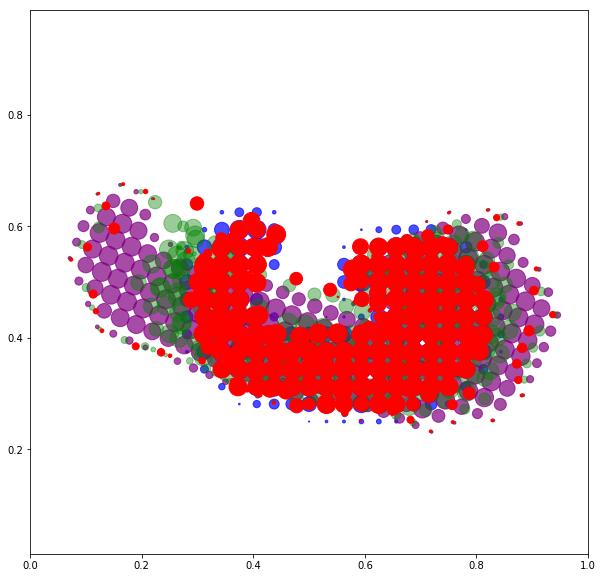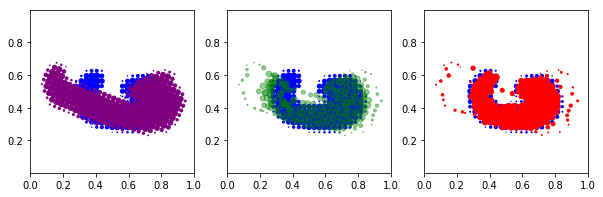Is this a desirable result?

Smoothing. Crucially, the "free particles" setting does not enforce any kind of smoothness on the deformation, and can induce tearings in the registration. Using a kernel norm as a fidelity, we thus observe left-out particles that lag behind because of the fidelity's vanishing gradients. Going further, we may alleviate this problem with Wasserstein-like fidelities... But even then, we may then observe mass splittings instead of, say, rotations when trying to register two star-shaped measures - try it with an "X" and a "+"!

To alleviate this problem, a simple idea is to smooth the displacement field. That is, to use a vector field

\begin{align} v(x)~=~\sum_{i=1}^N k(x-x_i)\,p_i ~=~ \big(k\star \sum_{i=1}^N p_i\,\delta_{x_i}\big)(x) \end{align}

to move around our Dirac masses, with $k$ a blurring kernel function - e.g. a Gaussian.

Regularization. Keep in mind that if the Fourier transform of $k$ is positive, any sampled vector field $v$ may be generated through such linear combinations. To enforce some kind of prior, we thus have to introduce an additional regularization term to our cost function.

From a theoretical point of view, using a positive kernel, the best penalty term is the dual kernel norm

\begin{align} \big\|\sum_{i=1}^N p_i\,\delta_{x_i}\big\|_k^2 ~=~ \big\langle \sum_{i=1}^N p_i\,\delta_{x_i}, k\star \sum_{i=1}^N p_i\,\delta_{x_i} \big\rangle ~=~\sum_{i,j=1}^N \langle p_i,p_j\rangle\,k(x_i-x_j) \end{align}

which can be rewritten as a Sobolev-like penalty on the vector field $v$: \begin{align} \big\|\sum_{i=1}^N p_i\,\delta_{x_i}\big\|_k^2 ~=~\iint_{\omega\in\mathbb{R}^2} \frac{|\widehat{v}(\omega)|^2}{\widehat{k}(\omega)}\,\text{d}\omega ~=~ \|v\|_{k}^{*2}. \end{align}

As we strive to optimize a cost that reads

\begin{align} \text{Cost}(p) ~~=~~ \lambda_1\,\text{Fidelity}\big(\sum \alpha_i \delta_{x_i+v_i}, \beta\big) ~+~ \lambda_2\,\big\|\sum p_i\,\delta_{x_i}\big\|_k^2, \end{align}

the algorithm will find a compromise between accuracy and stretching.

Kernel dot product. In practice, we use a sampled interpolator

\begin{align} \Phi^k_p~:~ x=(x_i)\in\mathbb{R}^{N\times2} ~\mapsto~ x~+~v~=~ x~+~ K_{xx} p, \end{align}

where $K$ is the kernel matrix of the $x_i$'s and $p$ is the momentum field encoded as an N-by-2 tensor.

Exercise 1: Implement a (linear) kernel registration module.

In :
run -i nt_solutions/measures_2/exo1

It  1:6.390. It  2:10.851. It  3:2.041. It  4:1.065. It  5:0.707.
It  6:0.452. It  7:0.335. It  8:0.287. It  9:0.232. It 10:0.177.
It 11:0.151. It 12:0.139. It 13:0.132. It 14:0.126. It 15:0.122.
It 16:0.119. It 17:0.115. It 18:0.114. It 19:0.113. It 20:0.113.
It 21:0.112. It 22:0.111. It 23:0.109. It 24:0.109. It 25:0.108.
It 26:0.108. It 27:0.108. It 28:0.108. It 29:0.107. It 30:0.107.
It 31:0.107. It 32:0.107. It 33:0.107. It 34:0.106. It 35:0.106.
It 36:0.106. It 37:0.106. It 38:0.105. It 39:0.105. It 40:0.105.
It 41:0.105. It 42:0.105. It 43:0.105. It 44:0.105. It 45:0.104.
It 46:0.104. It 47:0.105. It 48:0.104. It 49:0.103. It 50:0.103.
It 51:0.103. It 52:0.103. It 53:0.102. It 54:0.102. It 55:0.102.
It 56:0.102. It 57:0.102. It 58:0.102. It 59:0.102. It 60:0.102.
It 61:0.102. It 62:0.102. It 63:0.102. It 64:0.101. It 65:0.101.
It 66:0.101. It 67:0.101. It 68:0.101. It 69:0.101. It 70:0.101.
It 71:0.101. It 72:0.101. It 73:0.101. It 74:0.101. It 75:0.101.
It 76:0.101. It 77:0.100. It 78:0.100. It 79:0.100. It 80:0.100.
It 81:0.100. It 82:0.100. It 83:0.100. It 84:0.100. It 85:0.100.
It 86:0.100. It 87:0.100. It 88:0.100. It 89:0.100. It 90:0.100.
It 91:0.100. It 92:0.100. It 93:0.100. It 94:0.100. It 95:0.100.
It 96:0.100. It 97:0.100. It 98:0.100. It 99:0.100. It100:0.100.
It101:0.100. It102:0.100. It103:0.100. It104:0.100.
b'CONVERGENCE: REL_REDUCTION_OF_F_<=_FACTR*EPSMCH'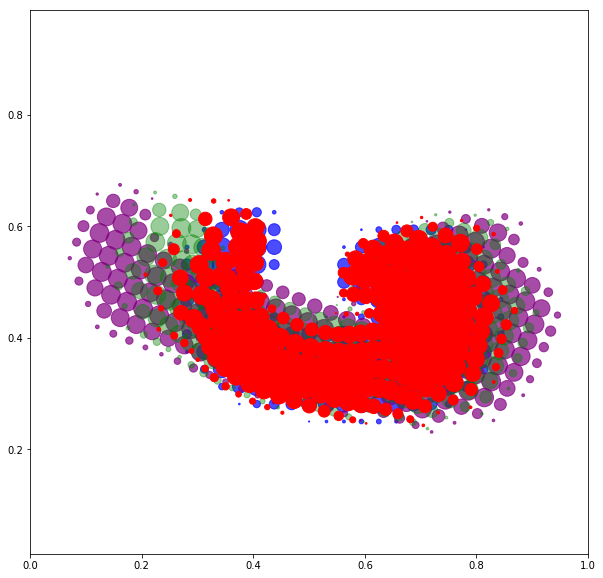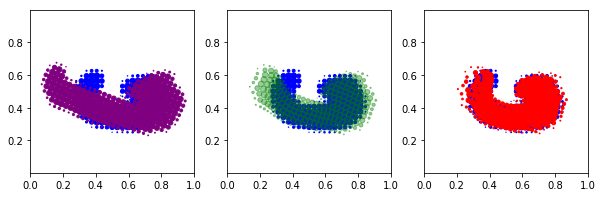<Figure size 432x288 with 0 Axes>

Smooth linear deformations have been extensively studied in the 90's, especially with Bookstein's Thin Plate Spline kernel:

\begin{align} \|v\|_{k}^{*2} ~~=~~ \iint_{\mathbb{R}^2} \|\partial^2_{xx} v(x)\|^2~ \text{d}x \end{align}

which allows us to retrieve affine deformations for free.

## B) A Riemannian model¶

Limits of the linear model. Assuming that the model $\Phi(\alpha)$ is close enough to $\beta$, we may use the matching momentum $p$ to characterize the deformation $\alpha\rightarrow\beta$. Its $k$-norm can be computed through

\begin{align} \big\|\sum_{i=1}^N p_i\,\delta_{x_i}\big\|_k ~~=~~ \sqrt{\langle p, K_{xx}p\rangle}_{\mathbb{R}^{N\times2}} \end{align}

and can be used as a "shape distance" $\text{d}_k(\alpha\rightarrow \beta)$ that penalizes tearings. Going further, we may compute the Fréchet mean of a population and perform kernel PCA on the momenta that link a mean shape to the observed samples.

Path distance in a space of measures. Unfortunately though, such an approach has a major drawback: The "kernel distance" is not symmetric. As it only makes use of the kernel matrix $K_{xx}$ computed on the source point cloud, it induces a bias which may be detrimental to statistical studies.

An elegant solution to this problem is to understand the kernel cost

$$\langle p, K_{xx} p\rangle ~=~ \langle v, K_{xx}^{-1} v\rangle$$

as a Riemannian, infinitesimal metric that penalizes small deformations. We may then look for trajectories

\begin{align} \alpha(\cdot)~:~t\in[0,1] ~\mapsto~ \alpha(t)~=~\sum_{i=1}^N \alpha_i\,\delta_{x_i(t)} \end{align}

such that $\alpha(0)=\alpha$ and minimize a cost

\begin{align} \text{Cost}(\alpha(\cdot)) ~~=~~ \lambda_1\,\text{Fidelity}(\alpha(1),\beta) ~+~ \lambda_2\,\int_0^1 \underbrace{\big\langle \dot{x}(t), K_{x(t),x(t)}^{-1}\,\dot{x}(t)\big\rangle}_{\|\dot{x}(t)\|_{x(t)}^2}\,\text{d}t \end{align}

with a regularization matrix that evolves along the path.

Momentum field. In practice, inverting the smoothing matrix is an ill-posed problem. We may thus parameterize the problem through the momentum field $(p_i(t))\in\mathbb{R}^{N\times 2}$ and write

\begin{align} \text{Cost}(\alpha(\cdot)) ~~=~~ \lambda_1\,\text{Fidelity}(\alpha(1),\beta) ~+~ \lambda_2\,\int_0^1 \big\langle p(t), K_{x(t),x(t)}\,p(t)\big\rangle\,\text{d}t, \end{align}

keeping in mind that $x(0)$ is given by $\alpha$ and that $\dot{x}=v=K_{x,x}p$

Exercise 2: Implement a path registration method, sampling the interval $[0,1]$ with 10 timesteps.

In :
run -i nt_solutions/measures_2/exo2

It  1:6.390. It  2:2.067. It  3:1.256. It  4:0.893. It  5:0.482.
It  6:0.326. It  7:0.258. It  8:0.232. It  9:0.175. It 10:0.156.
It 11:0.146. It 12:0.139. It 13:0.133. It 14:0.122. It 15:0.122.
It 16:0.119. It 17:0.116. It 18:0.114. It 19:0.111. It 20:0.111.
It 21:0.110. It 22:0.109. It 23:0.108. It 24:0.108. It 25:0.107.
It 26:0.106. It 27:0.105. It 28:0.104. It 29:0.103. It 30:0.103.
It 31:0.102. It 32:0.102. It 33:0.102. It 34:0.101. It 35:0.101.
It 36:0.100. It 37:0.100. It 38:0.100. It 39:0.100. It 40:0.100.
It 41:0.099. It 42:0.099. It 43:0.099. It 44:0.098. It 45:0.098.
It 46:0.098. It 47:0.097. It 48:0.097. It 49:0.097. It 50:0.097.
It 51:0.097. It 52:0.096. It 53:0.096. It 54:0.096. It 55:0.096.
It 56:0.096. It 57:0.096. It 58:0.096. It 59:0.096. It 60:0.096.
It 61:0.096. It 62:0.096. It 63:0.096. It 64:0.096. It 65:0.096.
It 66:0.095. It 67:0.095. It 68:0.095. It 69:0.095. It 70:0.095.
It 71:0.095. It 72:0.095. It 73:0.095. It 74:0.095. It 75:0.095.
It 76:0.095. It 77:0.095. It 78:0.095. It 79:0.095. It 80:0.095.
It 81:0.095. It 82:0.095. It 83:0.095. It 84:0.095. It 85:0.095.
It 86:0.095. It 87:0.095. It 88:0.095. It 89:0.095. It 90:0.095.
It 91:0.095. It 92:0.095. It 93:0.095. It 94:0.095. It 95:0.095.
It 96:0.094. It 97:0.094. It 98:0.094. It 99:0.094. It100:0.094.
It101:0.094. It102:0.094. It103:0.094. It104:0.094. It105:0.094.
It106:0.094. It107:0.094. It108:0.094. It109:0.094. It110:0.094.
It111:0.094. It112:0.094. It113:0.094. It114:0.094. It115:0.094.
It116:0.094. It117:0.094. It118:0.094. It119:0.094. It120:0.094.
It121:0.094.
b'CONVERGENCE: REL_REDUCTION_OF_F_<=_FACTR*EPSMCH'